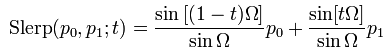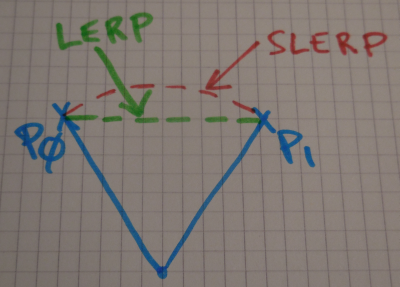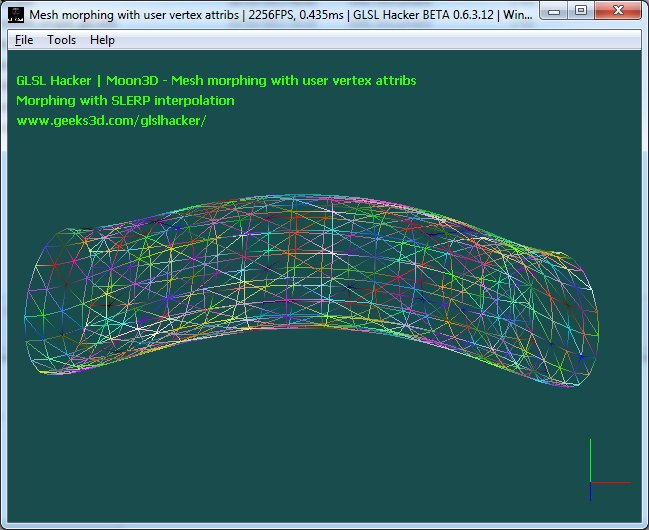# (GLSL) Simple Morph Target AnimationArticle index

## 1 – Overview

 Morph target animation is a technique that allows to deform a mesh using different deformed versions of the original mesh. This technique is used in character animation for example. The deformed versions are the morph targets (also called blend shapes). The deformation from one morph target to another one is done by interpolating the vertex positions. Here is a simple morph target animation technique that stores the vertex positions of the morph targets in the original mesh using vertex attribute arrays (glVertexAttribPointer in OpenGL). All morph targets must have the same number of vertices. All animation work is achieved in the vertex shader.

Here is the GLSL vertex shader in action: a morphing between a torus and a cylinder.

Demos (in Lua and GLSL) are coded with GLSL Hacker (Windows, OS X and Linux) and are available in the code sample pack in the moon3d/gl-320-mesh-morphing/ folder. It’s recommended to grab the latest DEV version (for GLSL Hacker and for the code sample pack).

As usual, the following GLSL shaders can be used in any OpenGL app that supports this kind of feature with minor changes only (the name of shader inputs).

In GLSL Hacker, a vertex has the following structure:

```vertex
{
vec4 Position;
vec4 Color;
vec4 Normal;
vec4 Tangent;
vec4 TexCoord0;
vec4 TexCoord1;
}
```

In the demos, we’re going to allocate new per-vertex attributes to store the positions of the morph-targets (In the Lua script, the allocation is done with gh_mesh.alloc_user_vertex_attribs()). For storing the positions of two morph-targets, we need two new vertex attributes: attrib0 and attrib1. The vertex has now the following structure:

```vertex
{
vec4 Position;
vec4 Color;
vec4 Normal;
vec4 Tangent;
vec4 TexCoord0;
vec4 TexCoord1;
vec4 Attrib0;
vec4 Attrib1;
}
```

## Linear interpolation

The first demo shows the morphing between a torus and a cylinder. In the following vertex shader, gxl3d_Position holds the torus positions, gxl3d_Attrib0 holds the vertex colors and gxl3d_Attrib1 holds the positions of the cylinder (vertex attrib arrays have been initialized in Lua with gh_mesh.set_user_vertex_attrib()). The uniform called time varies from 0.0 to 1.0. This vertex shader uses a linear interpolation (or lerp):

```#version 150
in vec4 gxl3d_Position;
in vec4 gxl3d_Attrib0;
in vec4 gxl3d_Attrib1;
out vec4 Vertex_Color;
uniform mat4 gxl3d_ModelViewProjectionMatrix;
uniform float time;
void main()
{
vec4 P;
P = gxl3d_Position + (gxl3d_Attrib1 - gxl3d_Position) * time;
gl_Position = gxl3d_ModelViewProjectionMatrix * P;
Vertex_Color = gxl3d_Attrib0;
}
```

The linear interpolation:

```P = gxl3d_Position + (gxl3d_Attrib1 - gxl3d_Position) * time;
```

can be rewritten using the GLSL built-in lerp function: mix():

```P = mix(gxl3d_Position, gxl3d_Attrib1, time);
```

## Smoothstep interpolation

The demo is also available with a better interpolation based to the smoothstep function. The following vertex shader shows smoothstep interpolation based on either the GLSL built-in smoothstep() function or on a direct implementation (SMOOTHSTEP define):

```#version 150
in vec4 gxl3d_Position;
in vec4 gxl3d_Attrib0;
in vec4 gxl3d_Attrib1;
out vec4 Vertex_Color;
uniform mat4 gxl3d_ModelViewProjectionMatrix;
uniform float time;
#define SMOOTHSTEP(x) ((x) * (x) * (3 - 2 * (x)))
void main()
{
//float alpha = SMOOTHSTEP(time);
float alpha = smoothstep(0.0, 1.0, time);
vec4 P = (gxl3d_Position * alpha) + (gxl3d_Attrib1 * (1 - alpha));
gl_Position = gxl3d_ModelViewProjectionMatrix * P;
Vertex_Color = gxl3d_Attrib0;
}
```

Or using the mix() function:

```P = mix(gxl3d_Position, gxl3d_Attrib1, smoothstep(.0,1.,time));
```

## Spherical linear interpolation

Another interesting interpolation method is the spherical linear interpolation (or slerp):Here is the vertex shader with SLERP interpolation:

```#version 150
in vec4 gxl3d_Position;
in vec4 gxl3d_Attrib0;
in vec4 gxl3d_Attrib1;
out vec4 Vertex_Color;
uniform mat4 gxl3d_ModelViewProjectionMatrix;
uniform float time;

vec4 Slerp(vec4 p0, vec4 p1, float t)
{
float dotp = dot(normalize(p0), normalize(p1));
if ((dotp > 0.9999) || (dotp<-0.9999))
{
if (t<=0.5)
return p0;
return p1;
}
float theta = acos(dotp * 3.14159/180.0);
vec4 P = ((p0*sin((1-t)*theta) + p1*sin(t*theta)) / sin(theta));
P.w = 1;
return P;
}

void main()
{
vec4 P = Slerp(gxl3d_Position, gxl3d_Attrib1, time);
gl_Position = gxl3d_ModelViewProjectionMatrix * P;
Vertex_Color = gxl3d_Attrib0;
}
```

The code sample pack also includes a demo that shows how to use two morph targets (a torus and a cylinder) to deform a sphere. Nothing new here, just the way to manage two morph-targets. In the following vertex shader, gxl3d_Position holds the sphere positions, gxl3d_Attrib0 holds the vertex colors, gxl3d_Attrib1 holds the positions of the first morph target (the torus) and gxl3d_Attrib2 holds the positions of the second morph target (the cylinder). Time uniform varies from 0.0 to 2.0.

```#version 150
in vec4 gxl3d_Position;
in vec4 gxl3d_Attrib0;
in vec4 gxl3d_Attrib1;
in vec4 gxl3d_Attrib2;
out vec4 Vertex_Color;
uniform mat4 gxl3d_ModelViewProjectionMatrix;
uniform float time;
void main()
{
vec4 P;

if (time < 1.0)
P = gxl3d_Position + (gxl3d_Attrib1 - gxl3d_Position) * time;
else
P = gxl3d_Attrib1 + (gxl3d_Attrib2 - gxl3d_Attrib1) * (time -1.0);

gl_Position = gxl3d_ModelViewProjectionMatrix * P;
Vertex_Color = gxl3d_Attrib0;
}
```Some references:

## One thought on “(GLSL) Simple Morph Target Animation”

1.pi

Line 18 of slerp:

float theta = acos(dotp * 3.14159/180.0);

Why convert dotp, aka cos(theta) from DEG -> RAD?

But cos(theta) isn’t in DEG. It isn’t an angle!

Isn’t the bracketing wrong?